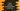# Java program to reverse an array without using an additional array## Java program to reverse an array without using an additional array:

In this post, we will learn how to reverse an array without using any additional array in Java. So, we will reverse the array in place, i.e. it will modify the given array and reverse its content.

### Algorithm:

The only way to do in place reverse is by iterating the array upto half. For each element it traverse, it will swap it with its exact opposite element from end.

So,

• Iterate all elements one by one up to (lenght/2).
• For each item it finds at index i, it will replace it with (length - i - 1)th item.

### Java program:

Let’s write down the complete Java program to understand it better:

``````package com.company;

import java.util.Arrays;

public class Main {
static int[] getReverseArray(int[] arr) {
int tempValue;

int arraySize = arr.length;

for (int i = 0; i < arraySize / 2; i++) {
tempValue = arr[i];
arr[i] = arr[arraySize - 1 - i];
arr[arraySize - 1 - i] = tempValue;
}

return arr;
}

public static void main(String[] args) {
System.out.println(Arrays.toString(getReverseArray(new int[]{1, 2, 3, 4, 5})));
System.out.println(Arrays.toString(getReverseArray(new int[]{1, 2, 3})));
System.out.println(Arrays.toString(getReverseArray(new int[]{1, 2, 3, 4, 5, 6, 7, 8, 9, 10})));
}
}``````

Here,

• getReverseArray method is used to reverse an array. It takes one integer array, reverse it in place and returns the new modified array.
• Inside this method,
• tempValue is an integer variable to hold a temporary value while swapping.
• arraySize is holding the length of the array.
• The for loop iterates the array elements one by one and iterates half of the elements.
• For each value it finds, it swaps it with the exact opposite element.
• Finally, after the for loop ends, it returns the same array.

In this example, we are checking three different arrays. If you run this program, it will print the below output:

``````[5, 4, 3, 2, 1]
[3, 2, 1]
[10, 9, 8, 7, 6, 5, 4, 3, 2, 1]``````